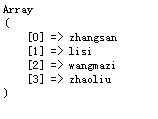# array_column函数低于PHP5.5之下的替代方法

 array_column 函数, 是能够根据多维数组中共有的一个键值来提取多维数组中属于这个键的值.例如下面的数组:\$test = array(``` 0 => array(　　　　 'id' => 11, 'name'=>'zhangsan' ), 1 => array(　　　　　'id' => 22, 'name'=> 'lisi' ), 2 => array(　　　　 'id' => 33, 'name'=> 'wangmazi' ), 3 => array(　　　　 'id' => 44, 'name'=> 'zhaoliu' ) );```要把他们提取到一个数组中, 那么就可以array_column()函数；`\$newNameArray = array_column(\$test, "name");`打印结果:如果要指定键值, 则`\$newNameArray = array_column(\$test, "name", "id");//\$test 操作的数组; 第一name 是 提取某个列的值; 第二个id表示用id来作为值得键`打印结果:方便好用, 但是只能在php5.5以上, 而且如果第二个参数  column_name  为 数字, 将返回空.下面的自定义函数很好的解决了这个问题：/**``` * array_column() // 不支持低版本; * 以下方法兼容PHP低版本 */ function _array_column(array \$array, \$column_key, \$index_key=null){ \$result = []; foreach(\$array as \$arr) { if(!is_array(\$arr)) continue; if(is_null(\$column_key)){ \$value = \$arr; }else{ \$value = \$arr[\$column_key]; } if(!is_null(\$index_key)){ \$key = \$arr[\$index_key]; \$result[\$key] = \$value; }else{ \$result[] = \$value; } } return \$result; }```参数与array_column() 相同, 用法也相同, 支持任何索引形式。